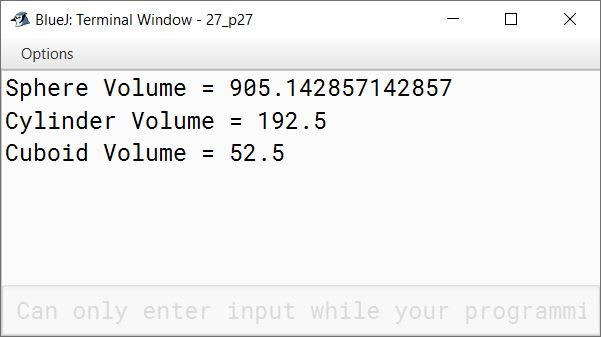# User Defined Methods

## Fill in the blanks

#### Question 1

A function can return only one value to its caller program.

#### Question 2

If a function does not return any value its return type is void.

#### Question 3

A function indicating the function name, return type along with function arguments is known as function header/prototype.

#### Question 4

The variables used to receive the values in function header are known as formal parameters.

#### Question 5

Method in a Java program resides in package.

#### Question 6

The entire function body is enclosed under curly brackets.

#### Question 7

The procedural function performs some actions without returning any output.

#### Question 8

A function contains header and body.

#### Question 9

Functions used with same name but different types of arguments are known as function overloading.

#### Question 10

A function that is called by itself in its body is known as recursive function.

## Tick the correct option

#### Question 1

The function which changes the state of an object is known as:

1. pure function
2. impure function ✓
3. replace function
4. none of the above

#### Question 2

Parameters used in function call statement are known as:

1. defined parameter
2. passed parameter
3. actual parameter ✓
4. formal parameter

#### Question 3

Parameters used in the function definition are called:

1. forward parameter
2. actual parameter
3. formal parameter ✓
4. none of the above

#### Question 4

The process of calling a function in such a way that the change in the formal arguments reflects on the actual parameter is known as:

1. call by reference ✓
2. call by value
3. call by method
4. none

#### Question 5

A function with many definitions is called:

1. multiple function
3. floating function
4. none

#### Question 6

A function may be associated with:

1. return ✓
2. call
3. promote
4. none

#### Question 7

Which of the following type can be used for a non-returnable function?

1. int
2. float
3. double
4. void ✓

#### Question 8

A function body is enclosed within a pair of:

1. { } ✓
2. [ ]
3. ( )
4. under a rectangular box

#### Question 9

A function is invoked through an:

1. object ✓
2. system
3. parameter
4. none

## Write TRUE or FALSE

#### Question 1

Calling and invoking a function is same.
True

#### Question 2

A method can use a single return statement.
True

False

#### Question 4

A function cannot be defined without parameters.
False

#### Question 5

A function body is enclosed within curly brackets.
True

#### Question 1

Define a function. What is meant by function prototype?

A function or a method is a sequence of statements grouped together and given a name. This group of statements can be called at any point in the program using its name to perform a specific task.
First line of function definition that tells about the type of value returned by the function and the number and type of arguments is called function prototype.

#### Question 2

What are the two ways of invoking functions?

Two ways of invoking functions are:

1. Pass by value.
2. Pass by reference.

#### Question 3

When a function returns the value, the entire function call can be assigned to a variable. Do you agree with the statement?

Yes, when a function returns a value, we can assign the entire function call to a variable. Below example illustrates the same:

```public class Example {

public int sum(int a, int b) {
int c = a + b;
return c;
}

public static void main(String args[]) {
Example obj = new Example();
int x = 2, y = 3;
int z = obj.sum(x, y);
System.out.println(z);
}
}```

#### Question 4

When a function is invoked how many values can be returned from the function?

A function can only return a single value.

#### Question 5

Debug the errors and rewrite the following function prototypes:

(a) int sum(x,y);

`int sum(int x, int y)`

(b) float product(a,int y);

`float product(float a, int y)`

(c) float operate(int x, float=3.4);

`float operate(int x, float y)`

(d) float sum(int x,y);

`float sum(int x, float y)`

#### Question 6

Write down the main function which calls the following function:

```int square(int a)
{
return(a*a);
}```
```public static void main(String args[]) {
int sq = square(4);
}```

#### Question 7

What happens when a function is passed by reference? Explain.

Pass by reference means that the arguments of the function are a reference to the original objects and not a copy. So any changes that the called function makes to the objects are visible to the calling function. Consider the below example:

```class PassByReferenceExample {
public void demoRef(int a[]) {
for (int i = 0; i < 5; i++) {
a[i] = i;
}
}

public static void main(String args[]) {
PassByReferenceExample obj = new PassByReferenceExample();
int arr[] = { 10, 20, 30, 40, 50 };
System.out.println("Before call to demoRef value of arr");
for (int i = 0; i < 5; i++) {
System.out.print(arr[i] + " ");
}
System.out.println();
obj.demoRef(arr);
System.out.println("After call to demoRef value of arr");
for (int i = 0; i < 5; i++) {
System.out.print(arr[i] + " ");
}
}
}```

The output of this program is:

``````Before call to demoRef value of arr
10 20 30 40 50
After call to demoRef value of arr
0 1 2 3 4
``````

Here `demoRef` changes the values of array `a` and these changes are reflected in the array in the main method as well.

#### Question 8

In what situation does a function return a value?

For a function to return a value, it should have a return type other than void in its function prototype and it should return a value of the corresponding type using the return statement in the function body.

#### Question 9

Differentiate between pure and impure functions.

Pure functionsImpure functions
Pure functions take objects and/or primitive data types as arguments but does not modify the objects.Impure functions change the state of received objects.
Pure functions doesn't have side effects.Impure functions have side effects.

#### Question 10

Write a function which is used to swap the values of two memory locations.

(a) by using a third variable.

```void swap(int a, int b) {
int c = a;
a = b;
b = c;
System.out.println("a = " + a + "\t" + "b = " + b);
}```

(b) without using a third variable.

```void swap(int a, int b) {
a = a + b;
b = a - b;
a = a - b;
System.out.println("a = " + a + "\t" + "b = " + b);
}```

#### Question 11

Differentiate between call by value and call by reference.

Call by valueCall by reference
Actual parameters are copied to formal parameters. Any changes to formal parameters are not reflected onto the actual parameters.Formal parameters refer to actual parameters. The changes to formal parameters are reflected onto the actual parameters.
All primitive data types are passed using Call by value.All reference data types like arrays and objects of classes are passed using Call by reference.

#### Question 12

What are the advantages of defining a method in a program?

Advantages of defining methods in a program are:

1. Methods help to manage the complexity of the program by dividing a bigger complex task into smaller, easily understood tasks.
2. Methods are useful in hiding the implementation details.
3. Methods help with code reusability.

#### Question 13

Function overloading is the process of defining functions/methods within a class, that have the same name but differ in the number and/or the data types of their arguments. Advantages of function overloading are:

1. Function overloading is one of the ways in which Java implements the object oriented concept of Polymorphism.
2. With Function overloading, programmers don't have to create and remember different names for functions doing the same thing for different data types.

#### Question 14

Define the following:

(a) Return data type

Return data type specifies the type of value that the method should return. It is mentioned before the method name in the method prototype. It can be any valid primitive or composite data type of Java. If no value is being returned, it should be void.

(b) Access specifier

Access specifiers determine the type of access to the method. It can be either public, private or protected.

(c) Parameter list

Parameter list is a comma-separated list of variables of a method along with their respective data types. The list is enclosed within a pair of parentheses. Parameter list can be empty if the method doesn't accept any parameters when it is called.

(d) Recursive function

A function that calls itself inside its body is called a Recursive function.

(e) Method signature

Method signature comprises of the method name and the data types of the parameters. For example, consider the below method:

```int sum(int a, int b) {
int c = a + b;
return c;
}```

Its method signature is:
`sum(int, int)`

#### Question 15

Explain the function of a return statement in Java programming.

A function returns a value through the return statement. Once a return statement is executed, the program control moves back to the caller function skipping the remaining statements of the current function if any. A function can have multiple return statements but only one of them will be executed. For example, consider the below method:

```int sum(int a, int b) {
int c = a + b;
return c;
}```

It uses a return statement to return a value of int type back to its caller.

#### Question 16

Differentiate between formal parameter and actual parameter.

Formal parameterActual parameter
Formal parameters appear in function definition.Actual parameters appear in function call statement.
They represent the values received by the called function.They represent the values passed to the called function.

#### Question 17

What is the role of the keyword void in declaring functions?

The keyword 'void' signifies that the function doesn't return a value to the calling function.

#### Question 18

If a function contains several return statements, how many of them will be executed?

A function can have multiple return statements but only one of them will be executed because once a return statement is executed, the program control moves back to the caller function skipping the remaining statements of the current function.

#### Question 20

How are the following data passed to a function?

• Primitive types
By value
• Reference types
By reference

## Give output of the following function definitions and also write what mathematical operations they carry out

#### Question 1

```void test1(int n)
{
for(int x=1; x<=n; x++)
if(n%x == 0)
System. out.println(x);
}```

if 12 is passed to n.

##### Output
``````1
2
3
4
6
12
``````
##### Explanation

This function finds the factors of n.

#### Question 2

```void test2(int a, int b)
{
while( a != b)
{
if ( a > b)
a = a — b;
else
a = b — a;
}
System.out.println(a);
}```

if 4 and 17 are passed to the function.

##### Output
``````Infinite Loop
``````
##### Explanation

Initial value of `a` is 4 and `b` is 17 as given in the question. As `a` and `b` are not equal, condition of while loop is true, first iteration starts. `a` is less than `b` so if condition is false, `a = b - a` is executed and `a` becomes `17 - 4 = 13`. Condition of while loop is true so second iteration starts. Again, if condition is false. This time `a` becomes `17 - 13 = 4`. Like this, the value of `a` keeps oscillating between 13 and 4 resulting in an infinite loop.

#### Question 3

```void test3(char c)
{
System.out.println( (int) c);
}```

if 'm' is passed to c.

##### Output
``````109
``````
##### Explanation

This function prints the ASCII code of its argument. When 'm' is passed to this function, its ASCII code which is 109 gets printed as the output.

#### Question 4

```void test4(String x, String y)
{
if(x.compareTo(y) > 0)
System.out.println(x);
else
System.out.println(y);
}```

if "AMIT" and "AMAN" are passed to the function.

##### Output
``````AMIT
``````
##### Explanation

The first differing characters of "AMIT" and "AMAN" are 'I' and 'A', respectively. So output of "AMIT".compareTo("AMAN") will be ASCII Code of 'I' - ASCII Code of 'A' ⇒ 73 - 65 ⇒ 8. The if condition is true so string x which is "AMIT" gets printed as the output.

## Solutions to Unsolved Java Programs

#### Question 1

Write a program in Java using a method Discount( ), to calculate a single discount or a successive discount. Use overload methods Discount(int), Discount(int,int) and Discount(int,int,int) to calculate single discount and successive discount respectively. Calculate and display the amount to be paid by the customer after getting discounts on the printed price of an article.
Sample Input:
Printed price: ₹12000
Successive discounts = 10%, 8%
= ₹(12000 - 1200)
= ₹(10800 - 864)
Amount to be paid = ₹9936

```import java.util.Scanner;

public class KboatSuccessiveDiscount
{
public void discount(int price) {
System.out.println("Amount after single discount = " + discount(price, 10));
System.out.println("Amount after successive discount = " + discount(price, 10, 8));
}

public double discount(int price, int d) {
double priceAfterDisc = price - price * d / 100.0;
return priceAfterDisc;
}

public double discount(int price, int d1, int d2) {
double priceAfterDisc1 = price - price * d1 / 100.0;
double priceAfterDisc2 = priceAfterDisc1 - priceAfterDisc1 * d2 / 100.0;
return priceAfterDisc2;
}

public static void main(String args[]) {

Scanner in = new Scanner(System.in);

System.out.print("Enter price: ");
int price = in.nextInt();

KboatSuccessiveDiscount obj = new KboatSuccessiveDiscount();

obj.discount(price);
}
}```
##### Output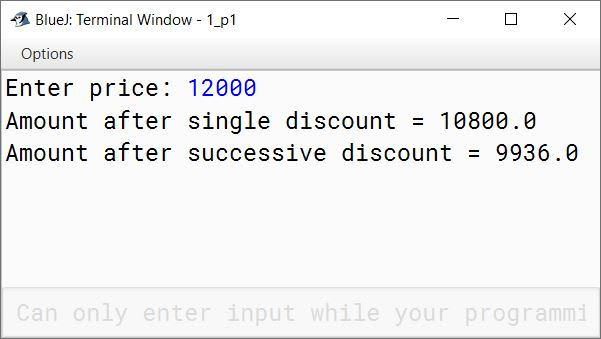#### Question 2

Write a program to input a number. Use a function int Armstrong(int n) to accept the number. The function returns 1, if the number is Armstrong, otherwise zero(0).
Sample Input: 153
Sample Output: 153 ⇒ 13 + 53 + 33 = 153
It is an Armstrong Number.

```import java.util.Scanner;

public class KboatArmstrongNumber
{
public int armstrong(int n) {

int num = n, cubeSum = 0;

while (num > 0) {
int digit = num % 10;
cubeSum = cubeSum + (digit * digit * digit);
num /= 10;
}

if (cubeSum == n)
return 1;
else
return 0;
}

public static void main(String args[]) {

Scanner in = new Scanner(System.in);
System.out.print("Enter Number: ");
int num = in.nextInt();

KboatArmstrongNumber obj = new KboatArmstrongNumber();
int r = obj.armstrong(num);

if (r == 1)
System.out.println(num + " is an Armstrong number");
else
System.out.println(num + " is not an Armstrong number");
}
}```
##### Output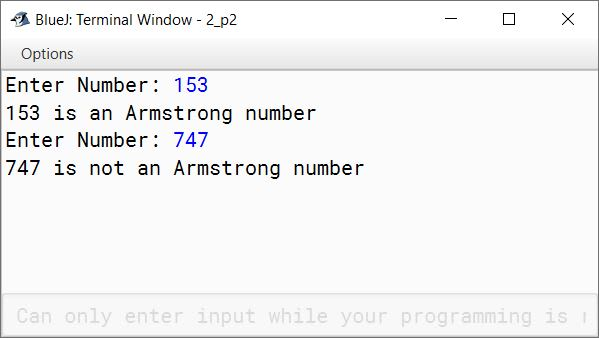#### Question 3

Write a program to input a number and check and print whether it is a 'Pronic' number or not. Use a function int Pronic(int n) to accept a number. The function returns 1, if the number is 'Pronic', otherwise returns zero (0).
(Hint: Pronic number is the number which is the product of two consecutive integers)
Examples:
12 = 3 * 4
20 = 4 * 5
42 = 6 * 7

```import java.util.Scanner;

public class KboatPronicNumber
{
public int pronic(int n) {

int isPronic = 0;

for (int i = 1; i <= n - 1; i++) {
if (i * (i + 1) == n) {
isPronic = 1;
break;
}
}

return isPronic;
}

public static void main(String args[]) {

Scanner in = new Scanner(System.in);
System.out.print("Enter the number to check: ");
int num = in.nextInt();

KboatPronicNumber obj = new KboatPronicNumber();
int r = obj.pronic(num);

if (r == 1)
System.out.println(num + " is a pronic number");
else
System.out.println(num + " is not a pronic number");

}
}```
##### Output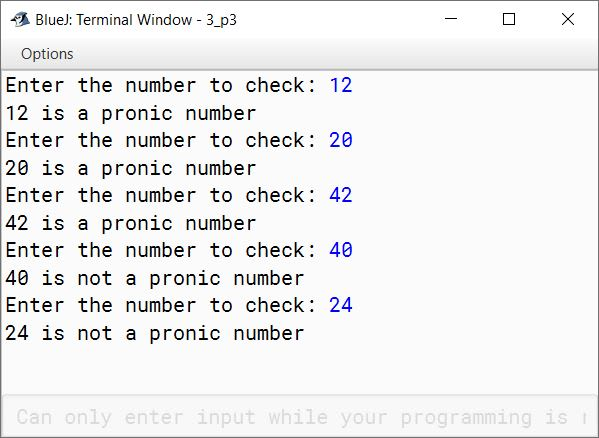#### Question 4

Write a program to enter a two digit number and find out its first factor excluding 1 (one). The program then find the second factor (when the number is divide by the first factor) and finally displays both the factors.
Hint: Use a non-return type function as void fact(int n) to accept the number.
Sample Input: 21
The first factor of 21 is 3
Sample Output: 3, 7
Sample Input: 30
The first factor of 30 is 2
Sample Output: 2, 15

```import java.util.Scanner;

public class KboatFactors
{
public void fact(int n) {

if (n < 10 || n > 99) {
System.out.println("ERROR!!! Not a 2-digit number");
return;
}

int i;
for (i = 2; i <= n; i++) {
if (n % i == 0)
break;
}

int sf = n / i;

System.out.println(i + ", " + sf);
}

public static void main(String args[]) {

Scanner in = new Scanner(System.in);

System.out.print("Enter number: ");
int num = in.nextInt();

KboatFactors obj = new KboatFactors();
obj.fact(num);
}
}```
##### Output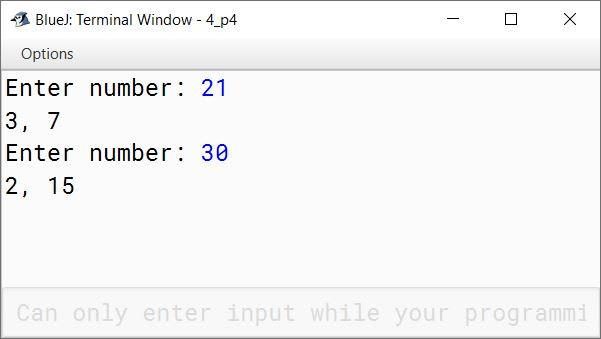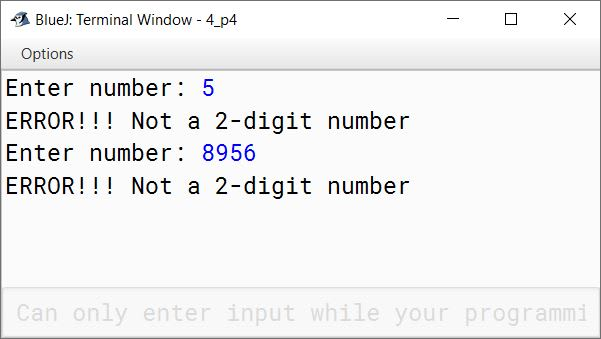#### Question 5

Write a function fact(int n) to find the factorial of a number n. Include a main class to find the value of S where:
S = n! / (m!(n - m)!)
where, n! = 1 x 2 x 3 x .......... x n

```import java.util.Scanner;

public class KboatFactorial
{
public long fact(int n) {

long f = 1;

for (int i = 1; i <= n; i++) {
f *= i;
}

return f;

}

public static void main(String args[]) {

KboatFactorial obj = new KboatFactorial();

Scanner in = new Scanner(System.in);
System.out.print("Enter m: ");
int m = in.nextInt();
System.out.print("Enter n: ");
int n = in.nextInt();

double s = (double)(obj.fact(n)) / (obj.fact(m) * obj.fact(n - m));
System.out.println("S=" + s);
}
}```
##### Output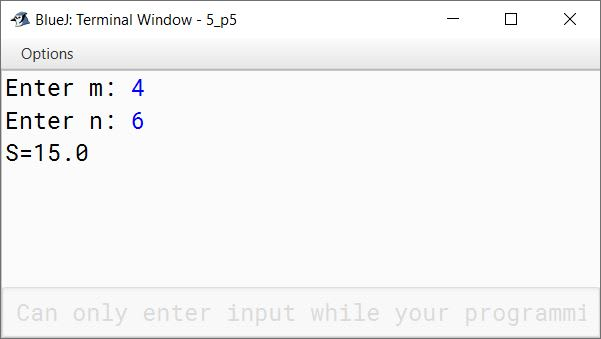#### Question 6

Write a program using a function called area() to compute area of the following:
(a) Area of circle = (22/7) * r * r
(b) Area of square= side * side
(c) Area of rectangle = length * breadth
Display the menu to display the area as per the user's choice.

```import java.util.Scanner;

{
public void area() {

Scanner in = new Scanner(System.in);

System.out.println("Enter a to calculate area of circle");
System.out.println("Enter b to calculate area of square");
System.out.println("Enter c to calculate area of rectangle");
char choice = in.next().charAt(0);

switch(choice) {
case 'a':
double r = in.nextDouble();
double ca = (22 / 7.0) * r * r;
System.out.println("Area of circle = " + ca);
break;

case 'b':
System.out.print("Enter side of square: ");
double side = in.nextDouble();
double sa = side * side;
System.out.println("Area of square = " + sa);
break;

case 'c':
System.out.print("Enter length of rectangle: ");
double l = in.nextDouble();
double b = in.nextDouble();
double ra = l * b;
System.out.println("Area of rectangle = " + ra);
break;

default:
System.out.println("Wrong choice!");
}
}
}```
##### Output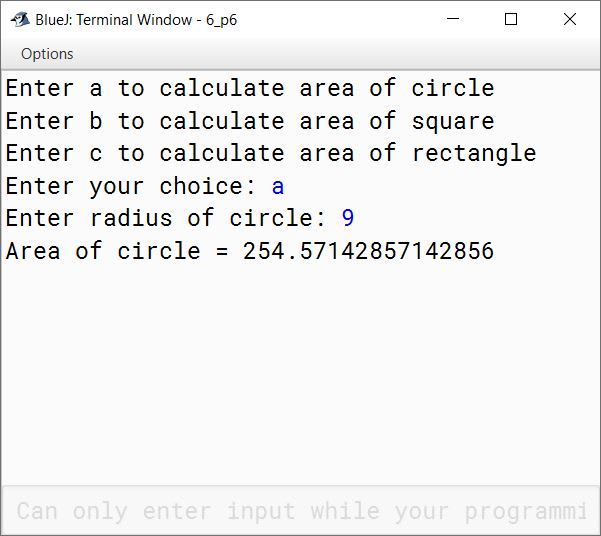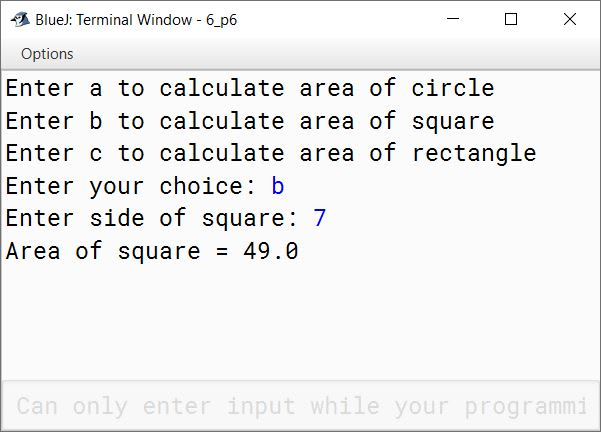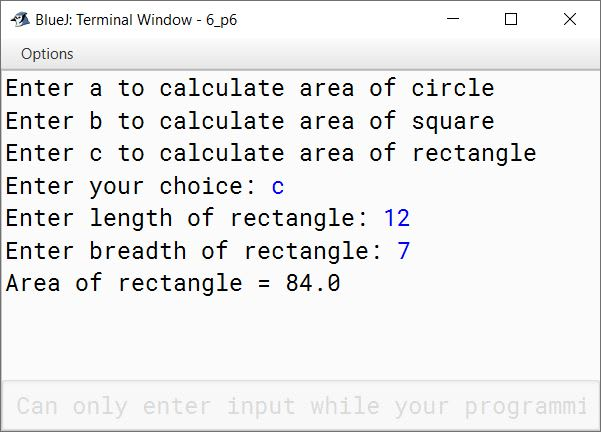#### Question 7

Write a program using method name Glcm(int,int) to find the Lowest Common Multiple (LCM) of two numbers by GCD (Greatest Common Divisor) of the numbers. GCD of two integers is calculated by continued division method. Divide the larger number by the smaller, the remainder then divides the previous divisor. The process is repeated till the remainder is zero. The divisor then results in the GCD.
LCM = product of two numbers / GCD

```import java.util.Scanner;

public class KboatGlcm
{

public void Glcm(int a, int b) {
int x = a, y = b;
while (y != 0) {
int t = y;
y = x % y;
x = t;
}

int lcm = (a * b) / x;

System.out.println("LCM = " + lcm);
}

public static void main(String args[]) {

Scanner in = new Scanner(System.in);
System.out.print("Enter first number: ");
int x = in.nextInt();
System.out.print("Enter second number: ");
int y = in.nextInt();

KboatGlcm obj = new KboatGlcm();
obj.Glcm(x, y);
}
}```
##### Output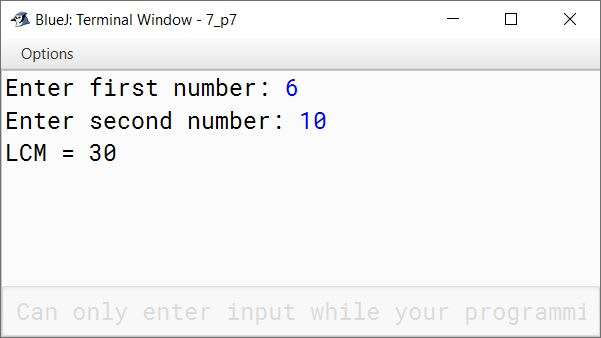#### Question 8

Write a program in Java to accept a word. Pass it to a function magic(String str). The function checks the string for the presence of consecutive letters. If two letters are consecutive at any position then the function prints "It is a magic string", otherwise it prints "It is not a magic string".
Sample Input: computer
Sample Output: It is not a magic string
Sample Input: DELHI
Sample Output: It is a magic string

```import java.util.Scanner;

public class KboatMagicString
{
public void magic(String str) {

boolean isMagicStr = false;
String t = str.toUpperCase();
int len = t.length();

for (int i = 0; i < len - 1; i++) {
if (t.charAt(i) + 1 == t.charAt(i + 1)) {
isMagicStr = true;
break;
}
}

if (isMagicStr)
System.out.println("It is a magic string");
else
System.out.println("It is not a magic string");
}

public static void main(String args[]) {

Scanner in = new Scanner(System.in);
System.out.print("Enter word: ");
String word = in.nextLine();

KboatMagicString obj = new KboatMagicString();
obj.magic(word);
}
}```
##### Output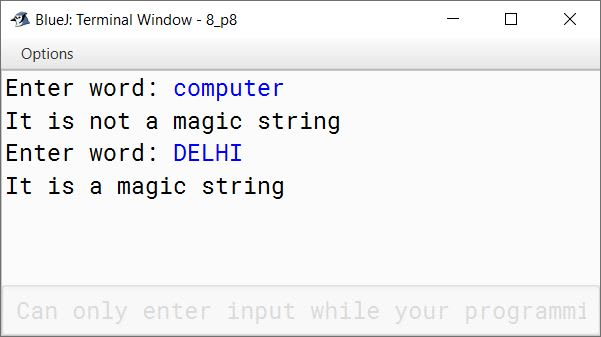#### Question 9

Write a program using a method Palin( ), to check whether a string is a Palindrome or not. A Palindrome is a string that reads the same from the left to right and vice versa.
Sample Input: MADAM, ARORA, ABBA, etc.

```import java.util.Scanner;

public class KboatStringPalindrome
{
public void palin() {

Scanner in = new Scanner(System.in);
System.out.print("Enter the string: ");
String s = in.nextLine();

String str = s.toUpperCase();
int strLen = str.length();
boolean isPalin = true;

for (int i = 0; i <  strLen / 2; i++) {
if (str.charAt(i) != str.charAt(strLen - 1 - i)) {
isPalin = false;
break;
}
}

if (isPalin)
System.out.println("It is a palindrome string.");
else
System.out.println("It is not a palindrome string.");
}
}```
##### Output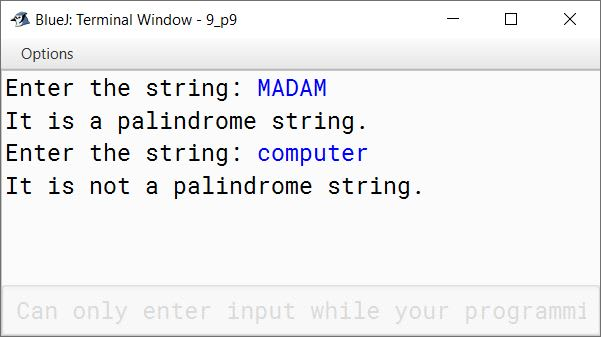#### Question 10

Write a program in Java to accept a String from the user. Pass the String to a function Display(String str) which displays the consonants present in the String.
Sample Input: computer
Sample Output:
c
m
p
t
r

```import java.util.Scanner;

public class KboatConsonants
{
public void display(String str) {

String t = str.toUpperCase();
int len = t.length();

for (int i = 0; i < len; i++) {
char ch = t.charAt(i);
if (ch != 'A' &&
ch != 'E' &&
ch != 'I' &&
ch != 'O' &&
ch != 'U') {
System.out.println(str.charAt(i));
}
}
}

public static void main(String args[]) {
Scanner in = new Scanner(System.in);
System.out.print("Enter string: ");
String s = in.nextLine();

KboatConsonants obj = new KboatConsonants();
obj.display(s);
}
}```
##### Output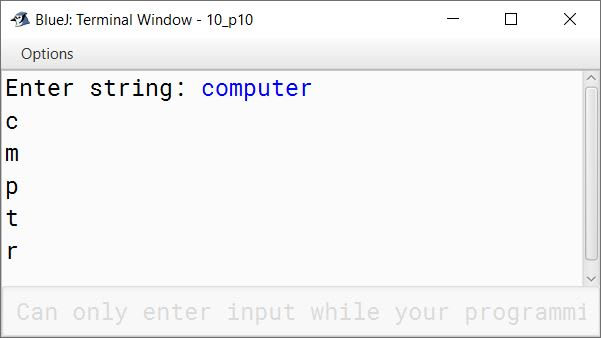#### Question 11

Write a program in Java to accept a String from the user. Pass the String to a function Change(String str) which displays the first character of each word after changing the case (lower to upper and vice versa).
Sample Input: Delhi public school
Sample Output:
d
P
S

```import java.util.Scanner;

public class KboatStringChange
{
public void change(String str) {

String t = " " + str;
int len = t.length();

for (int i = 0; i < len - 1; i++) {
if (t.charAt(i) == ' ') {
char ch = t.charAt(i+1);
if (Character.isUpperCase(ch))
ch = Character.toLowerCase(ch);
else if (Character.isLowerCase(ch))
ch = Character.toUpperCase(ch);
System.out.println(ch);
}
}
}

public static void main(String args[]) {
Scanner in = new Scanner(System.in);
System.out.print("Enter a string: ");
String s = in.nextLine();

KboatStringChange obj = new KboatStringChange();
obj.change(s);
}
}```
##### Output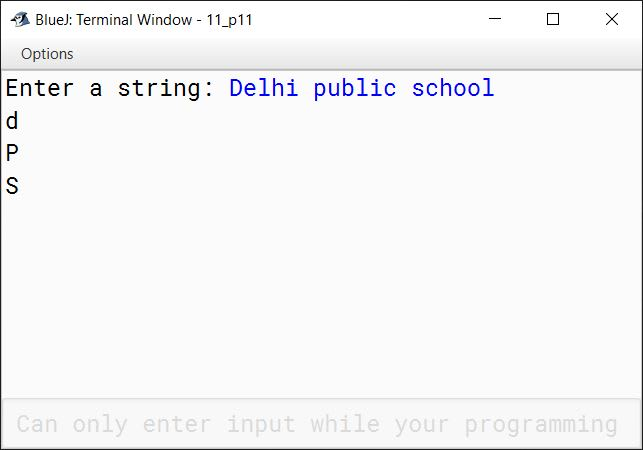#### Question 12

Write a program in Java to accept the name of an employee and his/her annual income. Pass the name and the annual income to a function Tax(String name, int income) which displays the name of the employee and the income tax as per the given tariff:

Annual IncomeIncome Tax
Up to ₹2,50,000No tax
₹2,50,001 to ₹5,00,00010% of the income exceeding ₹2,50,000
₹5,00,001 to ₹10,00,000₹30,000 + 20% of the amount exceeding ₹5,00,000
₹10,00,001 and above₹50,000 + 30% of the amount exceeding ₹10,00,000
```import java.util.Scanner;

public class KboatEmployeeTax
{
public void tax(String name, int income) {

double tax;
if (income <= 250000)
tax = 0;
else if (income <= 500000)
tax = (income - 250000) * 0.1;
else if (income <= 1000000)
tax = 30000 + ((income - 500000) * 0.2);
else
tax = 50000 + ((income - 1000000) * 0.3);

System.out.println("Name: " + name);
System.out.println("Income Tax: " + tax);
}

public static void main(String args[]) {
Scanner in = new Scanner(System.in);
System.out.print("Enter name: ");
String n = in.nextLine();
System.out.print("Enter annual income: ");
int i = in.nextInt();

KboatEmployeeTax obj = new KboatEmployeeTax();
obj.tax(n, i);
}
}```
##### Output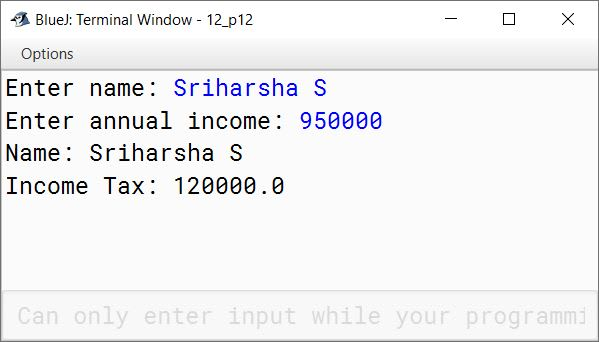#### Question 13

Write a program in Java to accept a String from the user. Pass the String to a function First(String str) which displays the first character of each word.
Sample Input : Understanding Computer Applications
Sample Output:
U
C
A

```import java.util.Scanner;

public class KboatFirstCharacter
{
public void first(String str) {
String t = " " + str;
int len = t.length();

for (int i = 0; i < len - 1; i++) {
if (t.charAt(i) == ' ') {
char ch = t.charAt(i + 1);
System.out.println(ch);
}
}

}

public static void main(String args[]) {
Scanner in = new Scanner(System.in);
System.out.print("Enter a string: ");
String s = in.nextLine();

KboatFirstCharacter obj = new KboatFirstCharacter();
obj.first(s);
}
}```
##### Output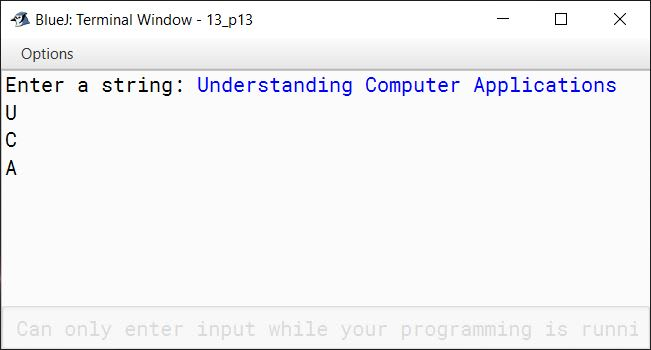#### Question 14

Write a program to accept 10 numbers in a Single Dimensional Array. Pass the array to a function Search(int m[], int ns) to search the given number ns in the list of array elements. If the number is present, then display the message 'Number is present' otherwise, display 'number is not present'.

```import java.util.Scanner;

public class KboatSDASearch
{
public void search(int m[], int ns) {
boolean found = false;
for (int i = 0; i < m.length; i++) {
if (m[i] == ns) {
found = true;
break;
}
}
if (found)
System.out.println("Number is present");
else
System.out.println("Number is not present");
}

public static void main(String args[]) {
Scanner in = new Scanner(System.in);
int arr[] = new int;
System.out.println("Enter 10 numbers");

for (int i = 0; i < arr.length; i++) {
arr[i] = in.nextInt();
}

System.out.print("Enter number to search: ");
int num = in.nextInt();

KboatSDASearch obj = new KboatSDASearch();
obj.search(arr, num);
}
}```
##### Output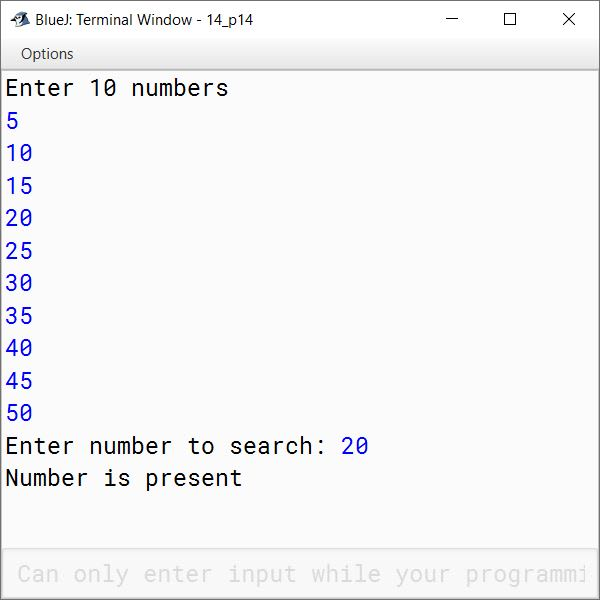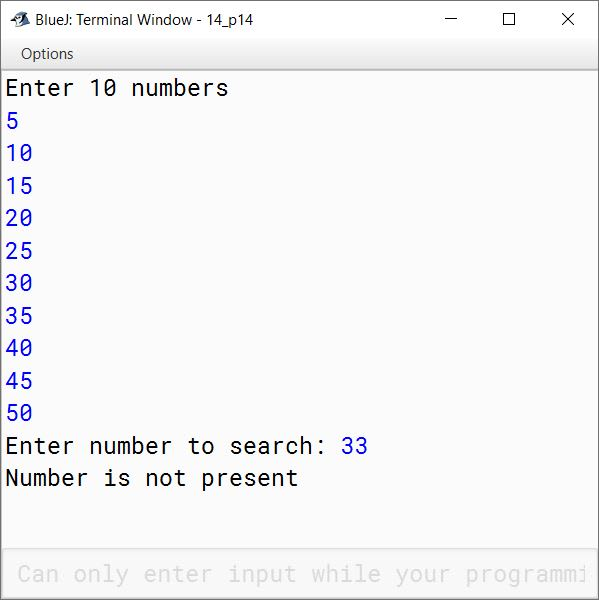#### Question 15

Write a class with the name Area using function overloading that computes the area of a parallelogram, a rhombus and a trapezium.

Formula:

Area of a parallelogram (pg) = base * ht

Area of a rhombus (rh) = (1/2) * d1 * d2
(where, d1 and d2 are the diagonals)

Area of a trapezium (tr) = (1/2) * ( a + b) * h
(where a and b are the parallel sides, h is the perpendicular distance between the parallel sides)

```import java.util.Scanner;

public class Area
{
public double area(double base, double height) {
double a = base * height;
return a;
}

public double area(double c, double d1, double d2) {
double a = c * d1 * d2;
return a;
}

public double area(double c, double a, double b, double h) {
double x = c * (a + b) * h;
return x;
}

public static void main(String args[]) {
Scanner in = new Scanner(System.in);
Area obj = new Area();

System.out.print("Enter base of parallelogram: ");
double base = in.nextDouble();
System.out.print("Enter height of parallelogram: ");
double ht = in.nextDouble();
System.out.println("Area of parallelogram = " + obj.area(base, ht));

System.out.print("Enter first diagonal of rhombus: ");
double d1 = in.nextDouble();
System.out.print("Enter second diagonal of rhombus: ");
double d2 = in.nextDouble();
System.out.println("Area of rhombus = " + obj.area(0.5, d1, d2));

System.out.print("Enter first parallel side of trapezium: ");
double a = in.nextDouble();
System.out.print("Enter second parallel side of trapezium: ");
double b = in.nextDouble();
System.out.print("Enter height of trapezium: ");
double h = in.nextDouble();
System.out.println("Area of trapezium = " + obj.area(0.5, a, b, h));
}
}```
##### Output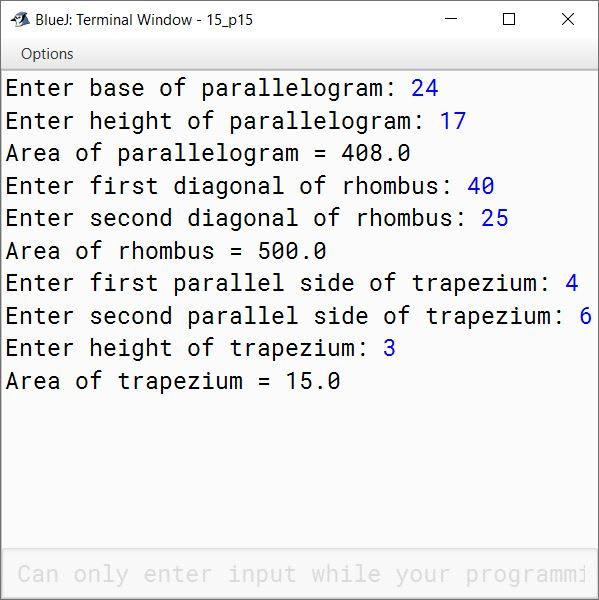#### Question 16

Write a class with the name Perimeter using function overloading that computes the perimeter of a square, a rectangle and a circle.

Formula:

Perimeter of a square = 4 * s

Perimeter of a rectangle = 2 * (l + b)

Perimeter of a circle = 2 * (22/7) * r

```import java.util.Scanner;

public class Perimeter
{
public double perimeter(double s) {
double p = 4 * s;
return p;
}

public double perimeter(double l, double b) {
double p = 2 * (l + b);
return p;
}

public double perimeter(int c, double pi, double r) {
double p = c * pi * r;
return p;
}

public static void main(String args[]) {
Scanner in = new Scanner(System.in);
Perimeter obj = new Perimeter();

System.out.print("Enter side of square: ");
double side = in.nextDouble();
System.out.println("Perimeter of square = " + obj.perimeter(side));

System.out.print("Enter length of rectangle: ");
double l = in.nextDouble();
double b = in.nextDouble();
System.out.println("Perimeter of rectangle = " + obj.perimeter(l, b));

double r = in.nextDouble();
System.out.println("Perimeter of circle = " + obj.perimeter(2, 3.14159, r));
}
}```
##### Output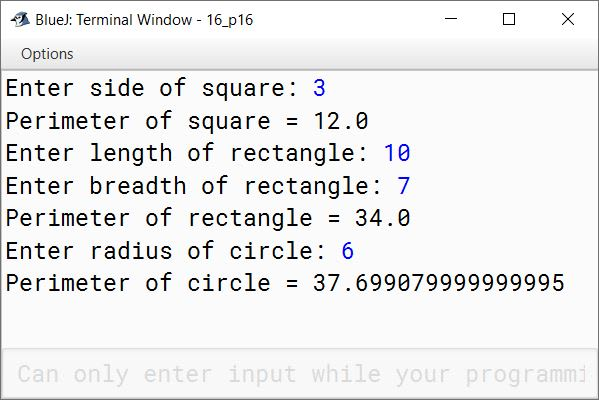#### Question 17

1. void display(String str, int p) with one String argument and one integer argument. It displays all the uppercase characters if 'p' is 1 (one) otherwise, it displays all the lowercase characters.
2. void display(String str, char chr) with one String argument and one character argument. It displays all the vowels if chr is 'v' otherwise, it displays all the alphabets.
```import java.util.Scanner;

{
void display(String str, int p) {

int len = str.length();

for (int i = 0; i < len; i++) {
char ch = str.charAt(i);
if (p == 1 && Character.isUpperCase(ch)) {
System.out.println(ch);
}
else if (p != 1 && Character.isLowerCase(ch)) {
System.out.println(ch);
}
}

}

void display(String str, char chr) {

int len = str.length();
for (int i = 0; i < len; i++) {
char ch = str.charAt(i);
ch = Character.toUpperCase(ch);
if (chr != 'v' && Character.isLetter(str.charAt(i)))
System.out.println(str.charAt(i));
else if (ch == 'A' ||
ch == 'E' ||
ch == 'I' ||
ch == 'O' ||
ch == 'U') {
System.out.println(str.charAt(i));
}
}

}

public static void main(String args[]) {
Scanner in = new Scanner(System.in);
System.out.print("Enter string: ");
String s = in.nextLine();

System.out.println("p=1");
obj.display(s, 1);
System.out.println("\np!=1");
obj.display(s, 0);
System.out.println("\nchr='v'");
obj.display(s, 'v');
System.out.println("\nchr!='v'");
obj.display(s, 'u');
}
}```
##### Output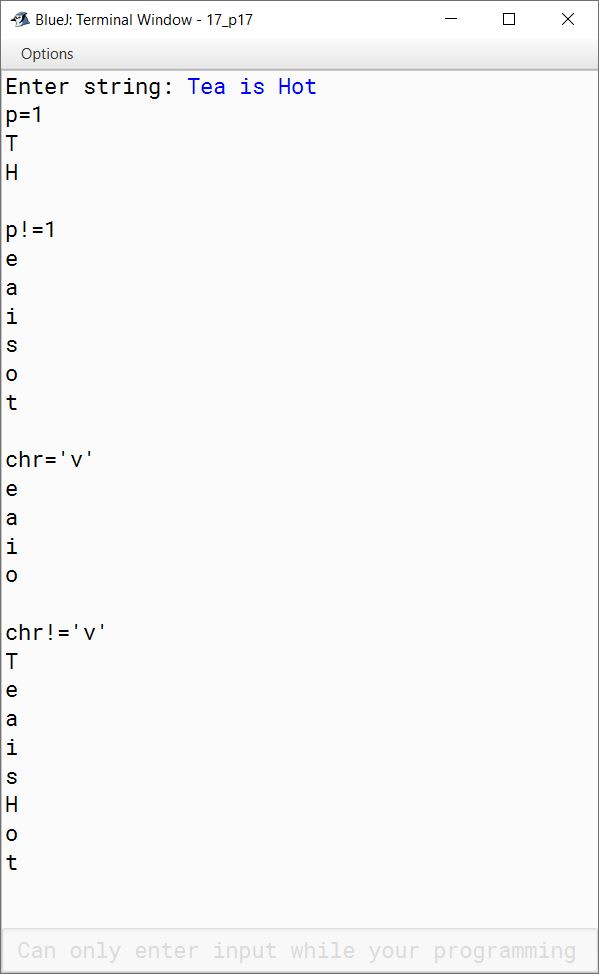#### Question 18

1. void calculate(int m, char ch) with one integer argument and one character argument. It checks whether the integer argument is divisible by 7 or not, if ch is 's', otherwise, it checks whether the last digit of the integer argument is 7 or not.
2. void calculate(int a, int b, char ch) with two integer arguments and one character argument. It displays the greater of integer arguments if ch is 'g' otherwise, it displays the smaller of integer arguments.
```import java.util.Scanner;

public class KboatCalculate
{
public void calculate(int m, char ch) {
if (ch == 's') {
if (m % 7 == 0)
System.out.println("It is divisible by 7");
else
System.out.println("It is not divisible by 7");
}
else {
if (m % 10 == 7)
System.out.println("Last digit is 7");
else
System.out.println("Last digit is not 7");
}
}

public void calculate(int a, int b, char ch) {
if (ch == 'g')
System.out.println(a > b ? a : b);
else
System.out.println(a < b ? a : b);
}

public static void main(String args[]) {
Scanner in = new Scanner(System.in);
KboatCalculate obj = new KboatCalculate();

System.out.print("Enter a number: ");
int n1 = in.nextInt();
obj.calculate(n1, 's');
obj.calculate(n1, 't');

System.out.print("Enter first number: ");
n1 = in.nextInt();
System.out.print("Enter second number: ");
int n2 = in.nextInt();
obj.calculate(n1, n2, 'g');
obj.calculate(n1, n2, 'k');

}
}```
##### Output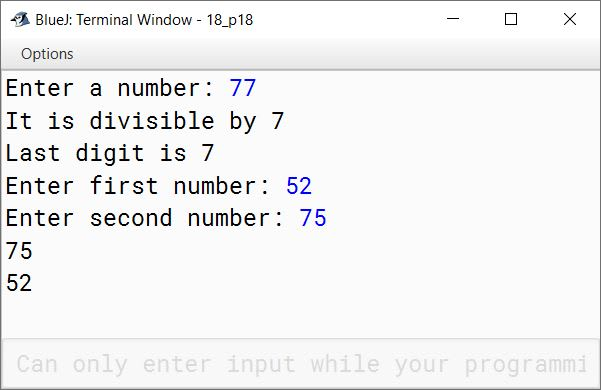#### Question 19

Write a menu driven program using a method Number() to perform the following tasks:

1. Accept a number from the user and display it in its Binary Equivalents.
For example:
Sample Input: (21)10
Sample Output: (10101)2
2. Accept a binary number from the user and display it in its Decimal Equivalents.
For example:
Sample Input: (11101)2
Sample Output: (29)10
```import java.util.Scanner;

public class KboatBinary
{
long decimal2Binary(int n) {
String str = "";
int t = n;
while (t > 0) {
int d = t % 2;
str = d + str;
t /= 2;
}

long b = Long.parseLong(str);
return b;
}

long binary2Decimal(long n) {
long decimal = 0;
int base = 1;
long t = n;
while (t > 0) {
int d = (int)(t % 10);
decimal += d * base;
base *= 2;
t /= 10;
}

return decimal;
}

void number() {
Scanner in = new Scanner(System.in);
System.out.println("Enter 1 to display number in Binary Equivalent");
System.out.println("Enter 2 to display number in Decimal Equivalent");
int c = in.nextInt();

switch (c) {
case 1:
System.out.print("Enter a decimal number: ");
int num = in.nextInt();
long binNum = decimal2Binary(num);
System.out.println("Binary Equivalent");
System.out.println(binNum);
break;

case 2:
System.out.print("Enter a binary number: ");
long ipNum = in.nextLong();
long decNum = binary2Decimal(ipNum);
System.out.println("Decimal Equivalent");
System.out.println(decNum);
break;

default:
System.out.println("Incorrect Choice");
break;
}
}
}```
##### Output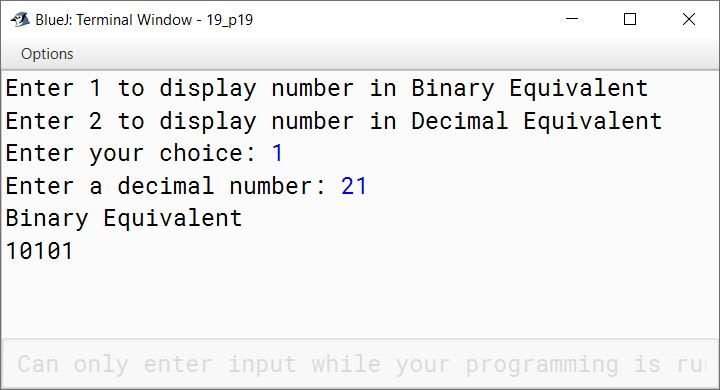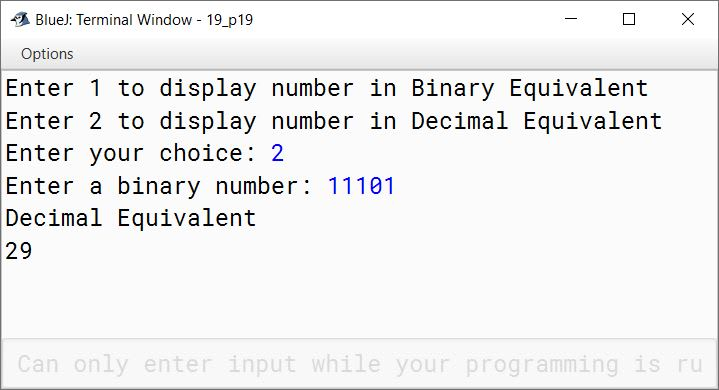#### Question 20

Design a class to overload a function manip() as follows:

1. void manip(String str, int p) with one String argument and one integer argument. It displays the characters of even positions of String, if p is an even number otherwise, it displays the characters of odd positions.
2. void manip(int a, char ch) with one integer argument and one character argument. It computes the square root of the integer arguments if ch is 's', else it computes the cube root of the integers.
```import java.util.Scanner;

public class KboatManip
{
public void manip(String str, int p) {

int len = str.length();
int s = 0;

if (p % 2 == 0) {
s = 1;
}

for (int i = s; i < len; i = i + 2) {
char ch = str.charAt(i);
System.out.println(ch);
}
}

public void manip(int a, char ch) {

double res = 0;

if (ch == 's') {
res = Math.sqrt(a);
}
else {
res = Math.cbrt(a);
}

System.out.println(res);
}

public static void main(String args[]) {
Scanner in = new Scanner(System.in);
KboatManip obj = new KboatManip();

System.out.print("Enter string: ");
String s = in.nextLine();
System.out.println("P is even");
obj.manip(s, 8);
System.out.println("\nP is odd");
obj.manip(s, 9);

System.out.print("Enter a number: ");
int num = in.nextInt();
System.out.println("\nch is 's'");
obj.manip(num, 's');
System.out.println("\nch is not 's'");
obj.manip(num, 'z');
}
}```
##### Output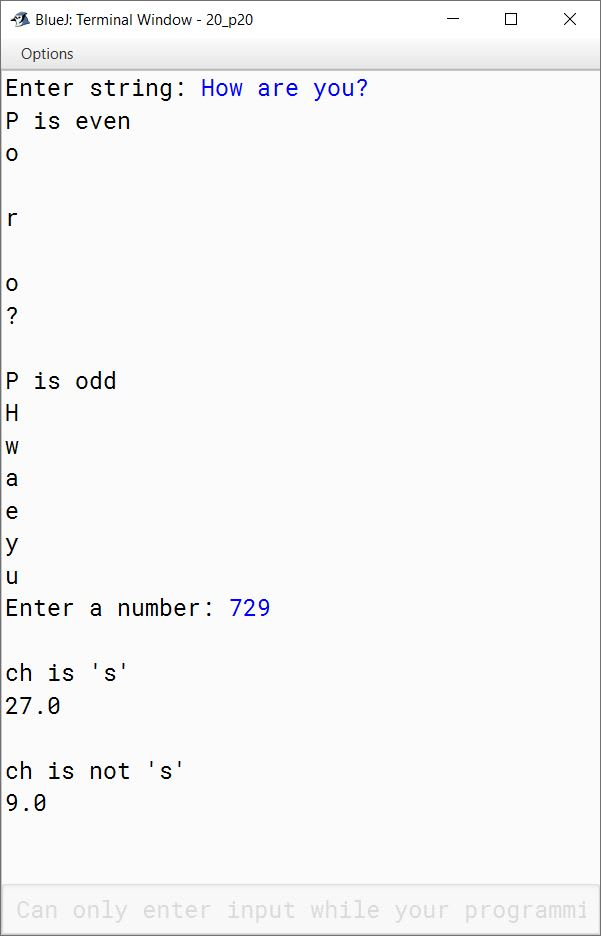#### Question 21

Design a class to overload a function compare( ) as follows:

1. void compare(int, int) — to compare two integers values and print the greater of the two integers.
2. void compare(char, char) — to compare the numeric value of two characters and print with the higher numeric value.
3. void compare(String, String) — to compare the length of the two strings and print the longer of the two.
```import java.util.Scanner;

public class KboatCompare
{
public void compare(int a, int b) {

if (a > b) {
System.out.println(a);
}
else {
System.out.println(b);
}

}

public void compare(char a, char b) {
int x = (int)a;
int y = (int)b;

if (x > y) {
System.out.println(a);
}
else {
System.out.println(b);
}

}

public void compare(String a, String b) {

int l1 = a.length();
int l2 = b.length();

if (l1 > l2) {
System.out.println(a);
}
else {
System.out.println(b);
}

}

public static void main(String args[]) {
Scanner in = new Scanner(System.in);
KboatCompare obj = new KboatCompare();

System.out.print("Enter first integer: ");
int n1 = in.nextInt();
System.out.print("Enter second integer: ");
int n2 = in.nextInt();
obj.compare(n1, n2);

System.out.print("Enter first character: ");
char c1 = in.next().charAt(0);
System.out.print("Enter second character: ");
char c2 = in.next().charAt(0);
in.nextLine();
obj.compare(c1, c2);

System.out.print("Enter first string: ");
String s1 = in.nextLine();
System.out.print("Enter second string: ");
String s2 = in.nextLine();
obj.compare(s1, s2);
}
}```
##### Output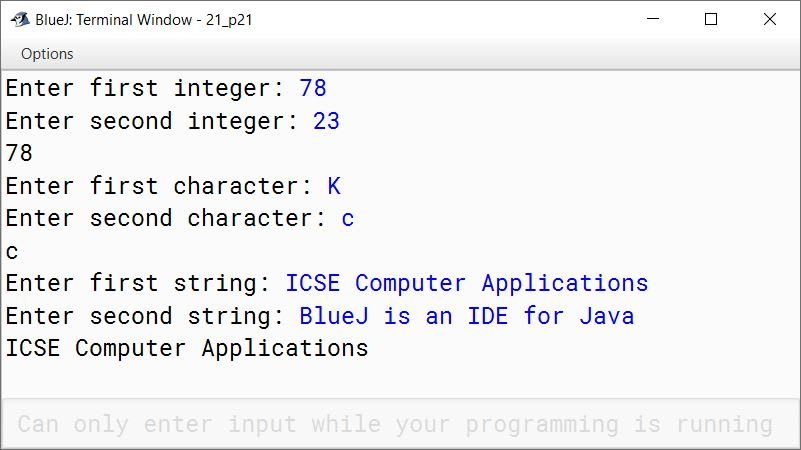#### Question 22

Design a class to overload a function polygon() as follows:

1. void polygon(int n, char ch) — with one integer and one character type argument to draw a filled square of side n using the character stored in ch.
2. void polygon(int x, int y) — with two integer arguments that draws a filled rectangle of length x and breadth y, using the symbol '@'.
3. void polygon() — with no argument that draws a filled triangle shown below:

Example:

1. Input value of n=2, ch = 'O'
Output:
OO
OO
2. Input value of x = 2, y = 5
Output:
@@@@@
@@@@@
3. Output:
*
**
***
```public class KboatPolygon
{
public void polygon(int n, char ch) {
for (int i = 1; i <= n; i++) {
for (int j = 1; j <= n; j++) {
System.out.print(ch);
}
System.out.println();
}
}

public void polygon(int x, int y) {
for (int i = 1; i <= x; i++) {
for (int j = 1; j <= y; j++) {
System.out.print('@');
}
System.out.println();
}
}

public void polygon() {
for (int i = 1; i <= 3; i++) {
for (int j = 1; j <= i; j++) {
System.out.print('*');
}
System.out.println();
}
}

public static void main(String args[]) {
KboatPolygon obj = new KboatPolygon();
obj.polygon(2, 'o');
System.out.println();
obj.polygon(2, 5);
System.out.println();
obj.polygon();
}
}```
##### Output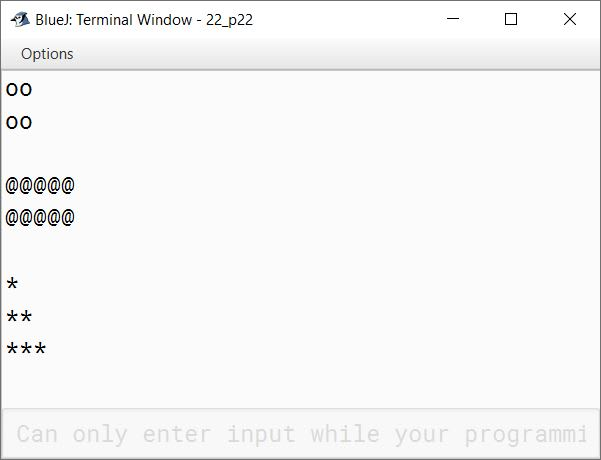#### Question 23

Design a class to overload a function series( ) as follows:

1. double series(double n) with one double argument and returns the sum of the series.
sum = (1/1) + (1/2) + (1/3) + .......... + (1/n)
2. double series(double a, double n) with two double arguments and returns the sum of the series.
sum = (1/a2) + (4/a5) + (7/a8) + (10/a11) + .......... to n terms
```public class KboatSeries
{
double series(double n) {
double sum = 0;
for (int i = 1; i <= n; i++) {
double term = 1.0 / i;
sum += term;
}
return sum;
}

double series(double a, double n) {
double sum = 0;
int x = 1;
for (int i = 1; i <= n; i++) {
int e = x + 1;
double term = x / Math.pow(a, e);
sum += term;
x += 3;
}
return sum;
}

public static void main(String args[]) {
KboatSeries obj = new KboatSeries();
System.out.println("First series sum = " + obj.series(5));
System.out.println("Second series sum = " + obj.series(3, 8));
}
}```
##### Output#### Question 24

Design a class to overload the function display(.....) as follows:

1. void display(int num) — checks and prints whether the number is a perfect square or not.
2. void display(String str, char ch) — checks and prints if the word str contains the letter ch or not.
3. void display(String str) — checks and prints the number of special characters present in the word str.

Write a suitable main( ) function.

```public class KboatDisplay
{
public void display(int num) {

double sroot = Math.sqrt(num);
double diff = sroot - Math.floor(sroot);

if (diff == 0)
System.out.println(num + " is a perfect square");
else
System.out.println(num + " is not a perfect square");

}

public void display(String str, char ch) {

int idx = str.indexOf(ch);

if (idx == -1)
else
System.out.println(ch + " found");

}

public void display(String str) {

int count = 0;
int len = str.length();

for (int i = 0; i < len; i++) {
char ch = str.charAt(i);
if (!Character.isLetterOrDigit(ch) &&
!Character.isWhitespace(ch)) {
count++;
}
}

System.out.println("Number of special characters = " + count);
}

public static void main(String args[]) {
KboatDisplay obj = new KboatDisplay();
obj.display(18);
obj.display("ICSE Computer Applications", 't');
obj.display("https://www.knowledgeboat.com/");
}
}```
##### Output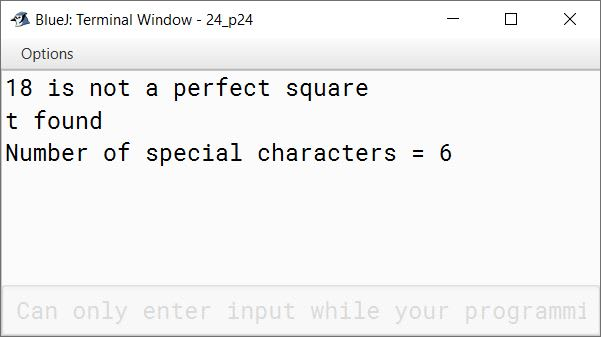#### Question 25

Design a class to overload the function display(.....) as follows:

1. void display(String str, char ch) — checks whether the word str contains the letter ch at the beginning as well as at the end or not. If present, print 'Special Word' otherwise print 'No special word'.
2. void display(String str1, String str2) — checks and prints whether both the words are equal or not.
3. void display(String str, int n) — prints the character present at nth position in the word str.

Write a suitable main() function.

```public class KboatWordCheck
{
public void display(String str, char ch) {

String temp = str.toUpperCase();
ch = Character.toUpperCase(ch);
int len = temp.length();

if (temp.indexOf(ch) == 0 &&
temp.lastIndexOf(ch) == (len - 1))
System.out.println("Special Word");
else
System.out.println("No Special Word");

}

public void display(String str1, String str2) {

if (str1.equals(str2))
System.out.println("Equal");
else
System.out.println("Not Equal");

}

public void display(String str, int n) {

int len = str.length();

if (n < 0 || n > len) {
System.out.println("Invalid value for argument n");
return;
}

char ch = str.charAt(n - 1);
System.out.println(ch);
}

public static void main(String args[]) {
KboatWordCheck obj = new KboatWordCheck();
obj.display("Tweet", 't');
obj.display("Tweet", "Massachusetts");
obj.display("Massachusetts", 8);
}
}```
##### Output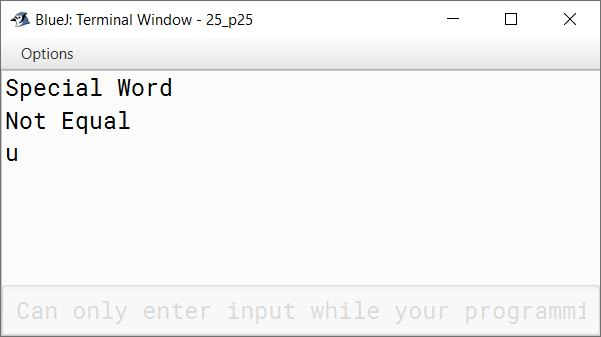#### Question 26

Design a class to overload a function Joystring( ) as follows:

1. void Joystring(String s, char ch1, char ch2) with one string argument and two character arguments that replaces the character argument ch1 with the character argument ch2 in the given String s and prints the new string.
Example:
Input value of s = "TECHNALAGY"
ch1 = 'A'
ch2 = 'O'
Output: "TECHNOLOGY"
2. void Joystring(String s) with one string argument that prints the position of the first space and the last space of the given String s.
Example:
Input value of s = "Cloud computing means Internet based computing"
Output:
First index: 5
Last Index: 36
3. void Joystring(String s1, String s2) with two string arguments that combines the two strings with a space between them and prints the resultant string.
Example:
Input value of s1 = "COMMON WEALTH"
Input value of s2 = "GAMES"
Output: COMMON WEALTH GAMES

(Use library functions)

```public class KboatStringOverload
{
public void joystring(String s, char ch1, char ch2) {
String newStr = s.replace(ch1, ch2);
System.out.println(newStr);
}

public void joystring(String s) {
int f = s.indexOf(' ');
int l = s.lastIndexOf(' ');
System.out.println("First index:  " + f);
System.out.println("Last index:  " + l);
}

public void joystring(String s1, String s2) {
String newStr = s1.concat(" ").concat(s2);
System.out.println(newStr);
}

public static void main(String args[]) {
obj.joystring("TECHNALAGY", 'A', 'O');
obj.joystring("Cloud computing means Internet based computing");
obj.joystring("COMMON WEALTH", "GAMES");
}
}```
##### Output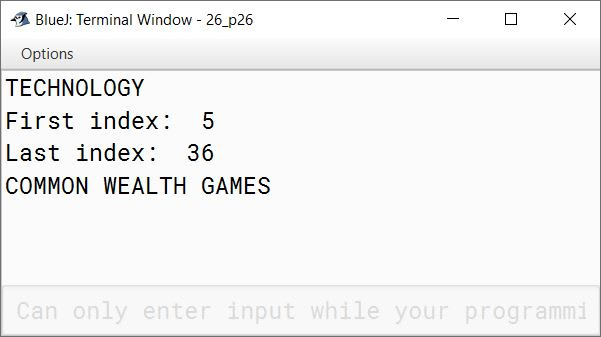#### Question 27

Design a class to overload a function volume( ) as follows:

1. double volume(double r) — with radius (r) as an argument, returns the volume of sphere using the formula:
V = (4/3) * (22/7) * r * r * r
2. double volume(double h, double r) — with height(h) and radius(r) as the arguments, returns the volume of a cylinder using the formula:
V = (22/7) * r * r * h
3. double volume(double 1, double b, double h) — with length(l), breadth(b) and height(h) as the arguments, returns the volume of a cuboid using the formula:
V = l*b*h ⇒ (length * breadth * height)
```public class KboatVolume
{
double volume(double r) {
double vol = (4 / 3.0) * (22 / 7.0) * r * r * r;
return vol;
}

double volume(double h, double r) {
double vol = (22 / 7.0) * r * r * h;
return vol;
}

double volume(double l, double b, double h) {
double vol = l * b * h;
return vol;
}

public static void main(String args[]) {
KboatVolume obj = new KboatVolume();
System.out.println("Sphere Volume = " +
obj.volume(6));
System.out.println("Cylinder Volume = " +
obj.volume(5, 3.5));
System.out.println("Cuboid Volume = " +
obj.volume(7.5, 3.5, 2));
}
}```
##### Output